## 3.8.2 KELDYSH Formulation

For certain applications in classical transport theory it is advantageous to write the BOLTZMANN equation as an integral equation, rather than an integro-differential equation. An analogous situation holds in quantum kinetics. Instead of working with the KADANOFF-BAYM equations (3.62) and (3.63), it may be useful to consider their integral forms. Historically, KELDYSH  derived his alternative form almost simultaneously and independently of KADANOFF and BAYM. However, the KELDYSH and KADANOFF-BAYM formalisms are equivalent.

By applying LANGRETH's rules to the DYSON equation (3.44) one obtains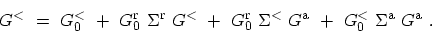(3.66)

For convenience a notation where a product of two terms is interpreted as a matrix product in the internal variables (space, time, etc.) has been used. One can proceed by iteration with respect to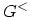. Iterating once, and regrouping the terms one obtains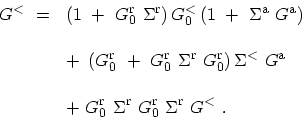(3.67)

The form of (3.67) suggests that infinite order iterations results in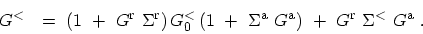(3.68)

Equation (3.68) is equivalent to KELDYSH's results. In the original work, however, it was written for another function,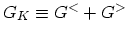. This difference is only of minor significance .

The first term on the right hand-side of (3.68) accounts for the initial conditions. One can show that this term vanishes for steady-state systems, if the system was in a non-interacting state in the infinite past . Thus, in many applications it is sufficient to only keep the second term.

Similar steps can be followed to obtain the kinetic equation for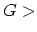. In integral form these equations can be written as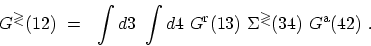(3.69)

The relation between the KELDYSH equation and the KADANOFF-BAYM equation is analogous to the relation between an ordinary differential equation plus a boundary condition and the corresponding integral equation.

M. Pourfath: Numerical Study of Quantum Transport in Carbon Nanotube-Based Transistors